## Working Capital Turnover Ratio

Working Capital Turnover Ratio Working Capital Turnover Ratio Working Capital Turnover Ratio- The working capital turnover ratio establishes a relationship between Revenue from Operations or Turnover and Working Capital. The …

## ISC ACCOUNTS Ratio Analysis MCQs with Solved Answers

ISC ACCOUNTS Ratio Analysis MCQs with Solved Answers ISC ACCOUNTS Ratio Analysis MCQs with Solved Answers (Question 1 to 5) 1. Net revenue from Operation: ₹30,00,000 Cost of Revenue from …

## Management Accounting MCQs with solved answers (Ratio analysis)

Management Accounting MCQs with solved answers (Ratio analysis) Management Accounting MCQs with solved answers (Ratio analysis) Management Accounting MCQs with solved answers (Ratio analysis) 1. The revenue from operations of …

## Management Accounting MCQs with solved answers (Ratio analysis)

Management Accounting MCQs with solved answers (Ratio analysis) Management Accounting MCQs with solved answers (Ratio analysis) Question 1-5 1. Ratio may be expressed in the: (a) Pure Ratio (b) So …

## Cash Flow From Operating Activities

Cash Flow From Operating Activities Cash Flow from Operating activities are the  cash flow from the principal revenue generating/Producing  activities of the enterprise that determine the net profit or loss. …

# P/V Ratio Formula

P/V Ratio Formula-

PROFIT-VOLUME RATIO (P/V Ratio) – Profit volume ratio refers to a ratio which establishes the relationship between contribution and sales.

This ratio 1s expressed as a percentage.

It is known as the P/V ratio, High P/V ratio indicates a high print provided there is no change in fixed cost.

The higher the contribution is, the greater wi be the P/V ratio.

A business can raise the P/V ratio by increasing selling price, optimum use of machines and men, increasing the efficiency of workers, etc.

This ratio is also known as Marginal Income Ratio, Contributions/Sales Ratio or, Variable Profit Ratio.

## Importance of P/V Ratio-

Profit volume ratio is a very important ratio. It helps in the calculation of many things such as…

1.To ascertain the variable cost at given sale

(ii) To calculate the Break-even pointii) To ascertain contribution at a given sale

Contribution= Sales × P/V Ratio

(iv) To take decisions in many areas

(v) To decide sales volume to achieve the desired profit

(vi) To have a comparative study of firms or products etc.

## Measures to Improve P/V Ratio-

1.By reducing variable costs

2.By increasing the selling price

3. By increasing the selling price at a rate higher than the variable costs.

4.By selling more products with higher PIN ratio.

### Also read : Golden Rules Of Accounting

P/V Ratio Formula-

### 1.When  the contribution and selling price are given in the question-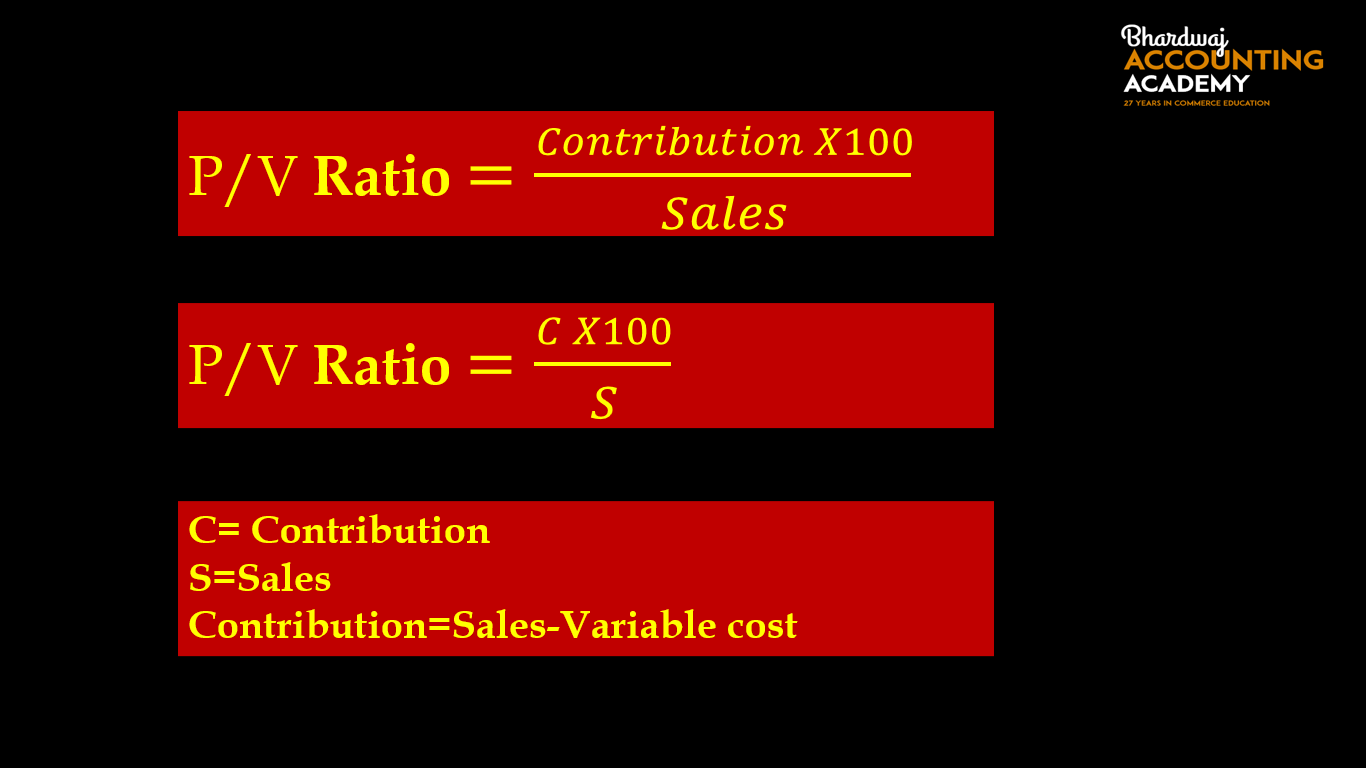The difference between selling price and variable cost is called contribution.

Or

Contribution= Fixed Cost + Profit

Or

Contribution= Fixed Cost – Loss

Or

Contribution= Sales × P/V Ratio

### 1.Example–

Find P/V Ratio from the following informations-

Selling price per unit : Rs. 200

Variable cost per unit: Rs. 120

Fixed Cost :Rs. 5,00,000

Solution-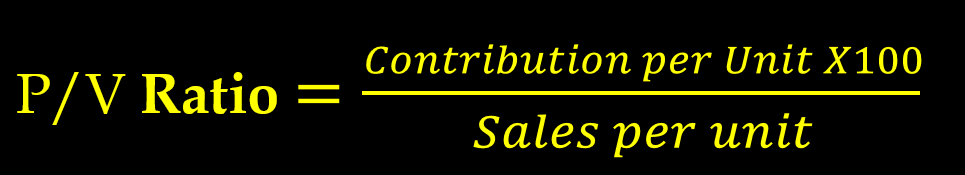Selling price per unit : Rs. 200

Variable cost per unit: Rs. 120

Contribution Per unit = 200-120

Contribution Per unit = 80

P/V Ratio= 80×100/200

P/V Ratio=40 %

2.Example

Find P/V Ratio from the following informations-

Selling price per unit : Rs. 500

Variable cost per unit: Rs. 400

Fixed Cost :Rs. 4,00,000

P/V Ratio Formula-

### 2.When   profit and sales for two financial years is  given in the question-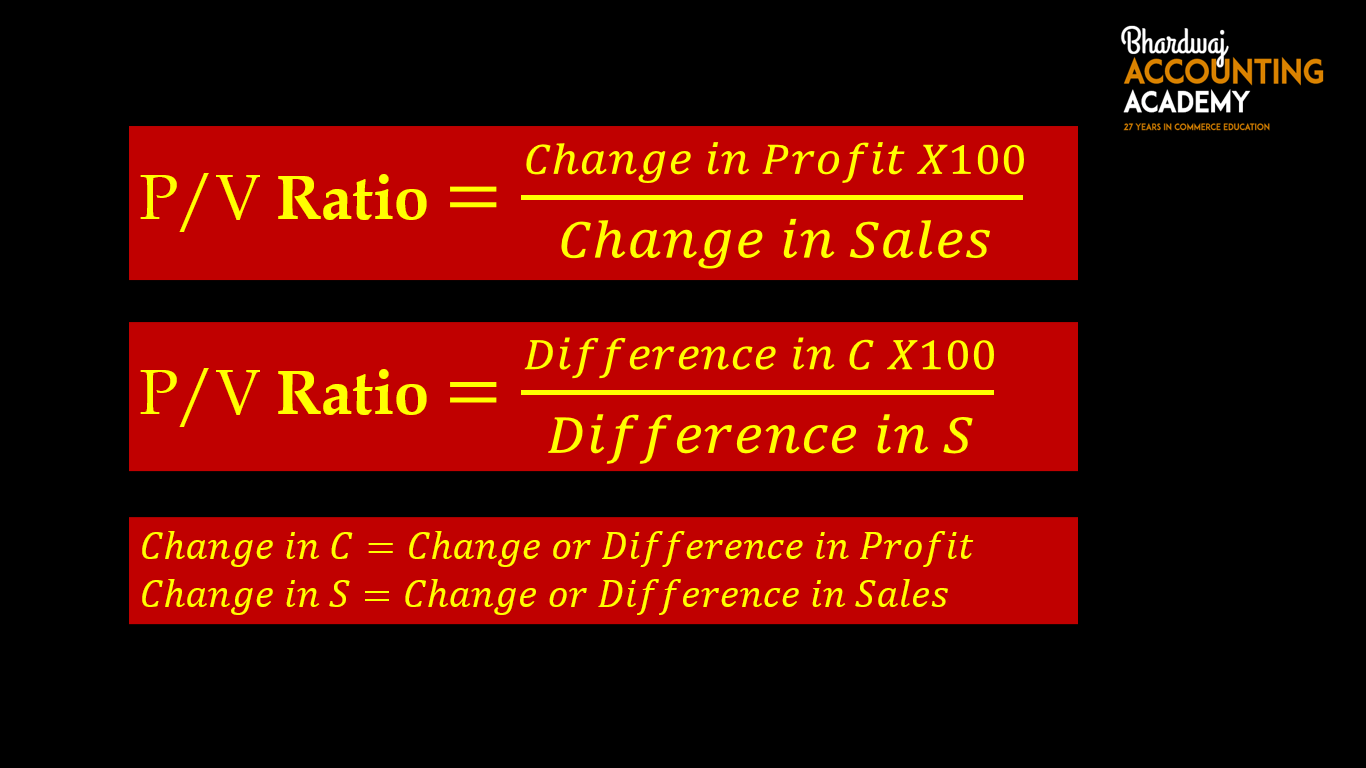### 3.Example– Find P/V Ratio from the following informations-

Sales :

2020-  Rs. 20,00,000

2021-  Rs. 24,00,000

Profit :

2020-  Rs. 3,00,000

2021-  Rs. 4,60,000

Solution-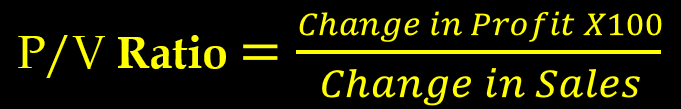Change in profit= 4,60,000-3,00,000

Change in profit=1,60,000

Change in Sales = 24,00,000-20,00,000

Change in Sales =4,00,000

P/V Ratio= 1,60,000 x 100/4,00,000

P/V Ratio=40 %

4.Example– Find P/V Ratio from the following informations-

Sales :

2020-  Rs. 50,00,000

2021-  Rs. 70,00,000

Profit :

2020-  Rs. 5,00,000

2021-  Rs. 6,80,000

P/V Ratio Formula-

### 3.When Profit, Fixed Cost and sales  is  given in the question-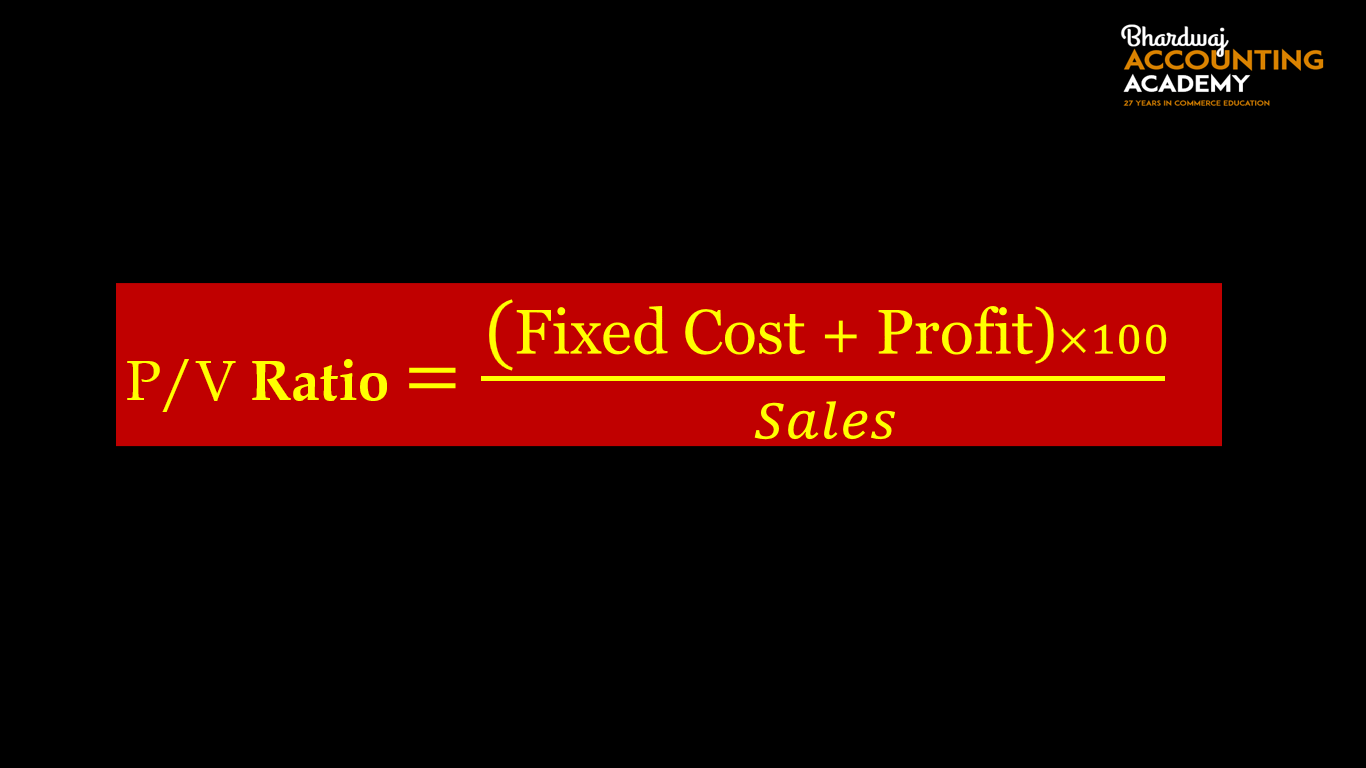### 5.Example–

Find P/V Ratio from the following informations-

Sales : Rs. 10,00,000

Profit : Rs. 2,00,000

Fixed Cost :Rs. 1,00,000

Solution-P/V Ratio= (1,00,000+2,00,000) × 100/10,00,000

P/V Ratio=3,00,000× 100/10,00,000

P/V Ratio=30 %

6.Example

Find P/V Ratio from the following informations-

Sales : Rs. 5,00,000

Profit : Rs. 1,00,000

Fixed Cost :Rs. 80,000

P/V Ratio Formula-

### 4.When Loss, Fixed Cost and sales  is  given in the question-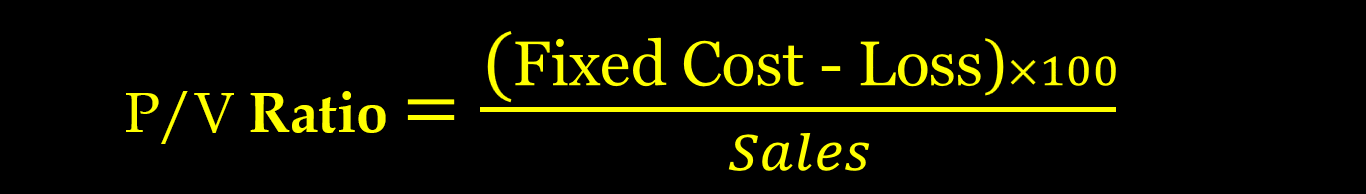### 7.Example–

Find P/V Ratio from the following informations-

Sales : Rs. 10,00,000

Loss : Rs. 2,00,000

Fixed Cost :Rs. 5,00,000

Solution-P/V Ratio= (5,00,000-2,00,000) × 100/10,00,000

P/V Ratio=3,00,000× 100/10,00,000

P/V Ratio=30 %

8.Example

Find P/V Ratio from the following informations-

Sales : Rs. 5,00,000

Loss : Rs. 50,000

Fixed Cost :Rs. 2,00,000